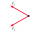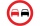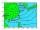# Fundamentals of Physics + addition - math problems

#### Number of problems found: 10

• Now isNow is 5°C morning. What will the temperature be if i. It rises by 12°C ii. It falls by 15°C
• Mass pointTwo equal forces of 30 Newtons act on a mass point. Find the magnitude of the resultant force if these forces form an angle of 42°.
• WeatherWeather. At 5:00 am, the temperature was 28°C, and at noontime, it rose by 9°C. By 4:00 pm, it dropped 3°C, and by nighttime, it was 4°C less. What is the room temperature this time?
• At noonAt noon today it was 6°C outside, but it has increased 4°C since then. What is the temperature outside now?
• Outdoor temperatureThe outdoor temperature was 8 degrees at midnight. The temperature declined 5 degrees during each of the next 3 hours. What was the temperature at 3am?
• OvertakingOn the direct road, the passenger car overtakes the slower bus by starting to overtake 20 meters from the bus and after passing it ahead of it again 20 meters away. The car overtakes at a steady speed of 72 km/h, the bus goes at a steady speed of 54 km/h.
• ParatrooperAfter the parachute is opened, the paratrooper drops to the ground at a constant speed of 2 m/s, with the sidewinding at a steady speed of 1.5 m/s. Find: a) the magnitude of its resulting velocity concerning the ground, b) the distance of his land from a
• Outside temperatureThe temperature outside was 57 degree Fahrenheit. During the next few hours it decreased by 18 degrees and then increased by 23 degrees. Find new temperature.
• Air pressureThe meteorological station measured the air pressure of 1060 hPa (hectopascals). In addition to measuring instruments, there is a residential house that has a flat roof with dimensions of 10 m and 28 m. What pressure force in MN (meganewtons) force the ai
• Two forcesTwo forces with magnitudes of 25 and 30 pounds act on an object at 10° and 100° angles. Find the direction and magnitude of the resultant force. Round to two decimal places in all intermediate steps and your final answer.

We apologize, but in this category are not a lot of examples.
Do you have an exciting math question or word problem that you can't solve? Ask a question or post a math problem, and we can try to solve it.

We will send a solution to your e-mail address. Solved examples are also published here. Please enter the e-mail correctly and check whether you don't have a full mailbox.

Please do not submit problems from current active competitions such as Mathematical Olympiad, correspondence seminars etc...

Fundamentals of Physics - math problems. Addition Problems.